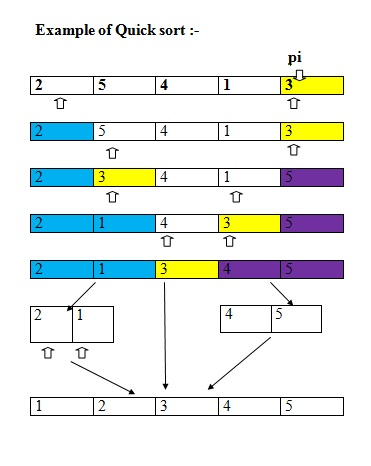# Quick Sort in C++ with Algorithm, Example

Quick Sort Algorithm with C++ Example: In this tutorial, we will learn about the quick sort algorithm and its implementation using the C++ program. By Amit Shukla Last updated : August 06, 2023

Quick sort is an efficient, general-purpose sorting algorithm. It was developed by British computer scientist Tony Hoare in 1959 and published in 1961. In quick sort we split the array into two parts and all the elements of one part is less than or equal to elements of other part for all possible indexes for both parts and if we sort these lines repeatedly then the entire array will be sorted. These are known as Divide and conquer approach.

## Description of quick sort

Pick a random pivot element pi , from, a partition a into the set of elements less than pi , the set of elements equal to pi , and the set of elements greater than pi and finally, recursively sort the first and third sets in this partition.

## Quick Sort Algorithm Function/Pseudo Code

```quickSort(array<T>& a)
{
quickSort(a, 0, a.length);
quickSort(array<T> & a, int i, int n)
{
if (n <= 1)
return;
T pi = a[i + rand() % n];
int p = i - 1, j = i, q = i + n;
while (j < q) {
int comp = compare(a[j], pi);
if (comp < 0) {
a.swap(j++, ++p); // move to beginning of array
}
else if (comp > 0) {
a.swap(j, --q); // move to end of array
}
else {
j++; // keep in the middle
}
}
}
}
// a[i..p]<pi, a[p+1..q-1]=pi, a[q..i+n-1]>pi
quickSort(a, i, p - i + 1);
quickSort(a, q, n - (q - i));
```

## Quick sort working flow

Consider the Quick sort working flow## C++ program to implement quick sort algorithm

```#include <iostream>
using namespace std;

void quicksort(int, int, int);
int partition(int, int, int);

int partition(int* a, int s, int e)
{
int piviot = a[e];
int pind = s;
int i, t;

for (i = s; i < e; i++) {
if (a[i] <= piviot) {
t = a[i];
a[i] = a[pind];
a[pind] = t;
pind++;
}
}

t = a[e];
a[e] = a[pind];
a[pind] = t;

return pind;
}

void quicksort(int* a, int s, int e)
{
if (s < e) {
int pind = partition(a, s, e);
quicksort(a, s, pind - 1);
quicksort(a, pind + 1, e);
}
}

int main()
{
int n;

cout << "Enter number of elements : " ;
cin >> n;

int a[n];

cout << "Enter the array : ";
for (int i = 0; i < n; i++) {
cin >> a[i];
}

quicksort(a, 0, n - 1);

cout << "Sorted array is : ";
for (int i = 0; i < n; i++) {
cout << a[i] << " ";
}

return 0;
}
```

## Output

```RUN 1:
Enter number of elements : 5
Enter the array : 10 20 5 15 10
Sorted array is : 5 10 10 15 20

RUN 2:
Enter number of elements : 10
Enter the array : 40 30 20 50 60 70 80 90 10 11
Sorted array is : 10 11 20 30 40 50 60 70 80 90
```